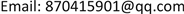VRP问题，遗传算法，多层编码，交通运输，物流，调度，路径优化, VRP Problem Genetic Algorithm Multi-Layer Coding Transportation Logistics Dispatching Path Optimization

1北京理工大学珠海学院工业自动化学院，广东 珠海

2华南理工大学土木与交通学院，广东 广州1. 引言1.1. 选题背景

1.2. 国内外研究现状

2. 车辆调度优化问题分析2.1. 多配送中心车辆调度问题的数学模型

①每条配送路径上各需求商的需求量之和不超过车辆的载重量；

②每条配送路径的长度不超过车辆一次配送的最大行驶距离；

③每个需求商的需求必须满足，且只能由一台车辆送货。

min Z = ∑ h = 1 H { ∑ k = 1 K h [ ∑ i = 1 n h k d r h k ( i − 1 ) r h k i + d r h k n h k r h k 0 ∗ s i g n ( n h k ) ] } (1)

s . t .       ∑ i = 1 n h i q h r h k i ≤ Q h k (2)

d r h k ( i − 1 ) r h k i + d r h k n h k r h k 0 ∗ s i g n ( n h k ) ≤ D h k (3)

0 ≤ n k ≤ L h (4)

∑ k = 1 K h n h k = L h (5)

∑ h = 1 H L h = M (6)

R n k = { r n k i | r n k i ∈ { 1 , 2 , ⋯ , L h } , i = 1 , 2 , ⋯ , n h k } (7)

R h k 1 ∩ R h k 2 = ∅ ,   ∀ h k 1 ≠ h k 2 (8)

s i g n ( n h k ) = { 1       n h k ≥ 1 0         其 他 (9)

2.2. 遗传算法

3. 车辆调度优化问题的建模3.1. 遗传算法解决问题的基本思想

3.2. VRP问题的影响因素分析

1) 运输距离；2) 运输环节；3) 运输相关的运营成本；4) 货物数量

1) 与时间相关的因素：a) 货物的运到期限，b) 货物的在途时间；2) 与交货质量相关的因素；3) 线路状态；4) 信息化管理水平。

3.3. 影响因素的确定以及评价标准

1) 建立递阶层次结构模型；

2) 构造出各层次中的所有判断矩阵；

3) 层次单排序及一致性检验；

4) 层次总排序及一致性检验。

Analytic hierarchy importance reference tabl

1Ci与Cj的影响程度相同
3Ci比Cj的影响程度稍强
5Ci比Cj的影响程度强
7Ci与Cj的影响程度明显的强
8Ci与Cj的影响程度绝对的强
2，4，6，8Ci与Cj的影响介于上述相邻等级之间
1, 1/2, 1/3, …, 1/9反过来，Ci与Cj之比aji = 1/aij

Hierarchical analysis importance tabl
i/j运输距离货物数量时间线路状态运输环节交货质量资金流转信息化管理水平

weight vector: 0.37721 0.1619 0.28694 0.082043 0.024833 0.025908 0.015824 0.025346

CI = 按照各比例重要度，以及影响水平，本文选取运输距离，货物数量(重量)，货物的在途时间，线路状态(对道路的破坏水平)四个指标进行影响因子的构建：

c i j = α ⋅ s + β ⋅ m + γ ⋅ t + δ ⋅ d       其 中 ( α + β + γ + δ = 1 ) (10)

4. 多层编码遗传算法解决VRP问题的实现

4.1. 模型构建

1) 供应集 M = { m 1 , m 2 , ⋯ , m m } ，mj表示第j个需求点， j = 1 , 2 , ⋯ , m 。

2) 需求集 P = { p 1 , p 2 , ⋯ , p n } ，mj表示第i个供应点， i = 1 , 2 , ⋯ , n 。

3) 路径序列集 O P = { o p 1 , o p 2 , ⋯ , o p n } ， o p i = { o p i 1 , o p i 2 , ⋯ , o p i k } ，表示供应商车辆所运送对应物资opi的运输批次。

4) 可提供相关所需物资的供应商的集合表示为

O P M = { o p i 1 , o p i 2 , ⋯ , o p i k }

5) 时间矩阵T， t i j ∈ T ，表示第i个供应商pi第j批次到达目的地的时间。

6) 代价矩阵C， c i j ∈ C ，表示第i个供应商pi第j批次到到达目的地的各方面代价总和。

4.2. 算法实现

1) 个体编码

[2 4 3 1 1 2 3 4 2 1 3 3 2 2 1 3]

2) 适应度值

3) 选择操作

p i ( i ) = Fitness ( i ) / ∑ i − 1 n Fitness (i)

4) 交叉操作

5) 变异操作

6) 计算交叉以及编译操作后适应度(即时间代价)值。

4.3. 算例4.3.1. 配送案例

Supply and demand materials supply and demand correspondence tabl

Transport final cost lis

C i j = 30 × 0.4154 + 10 × 0.1703 + 3 × 0.3159 + 1 × 0.098

4.3.2. 配送方案验证

5. 结论

1) 对于物流运输中多配送中心，构建了相应数学模型进行描述。

2) 应用改进的多层编码遗传算法加入多影响因子构建了一个解决多配送中心的车辆调度及路径规划问题的方法。

3) 通过实际案例需求，构建了可视化表格进行问题描述，应用所研究的方法，证实可以得到一个完整的全局代价最小的路径规划网络。